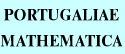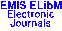PORTUGALIAEMATHEMATICA Vol. 56, No. 3, pp. 257-263 (1999)

Next Article

Contents of this Issue

Other Issues

ELibM Journals

ELibM Home

EMIS Home

## Primitive Quadratics Reflected in $B_{2}$-Sequences

### Bernt Lindström

Department of Mathematics, Royal Institute of Technology,
S-100 44 Stockholm - SWEDEN

Abstract: A $B_{2}$-sequence of positive integers $a_{1},a_{2},...,a_{r}$ has the property that the sums $a_{i}+a_{j}$, $1\le i\le j\le r$, are different. R.C. Bose and S. Chowla have proved the existence of $B_{2}$-sequences of size $r=q$, a power of a prime $p$, such that the sums $a_{i}+a_{j}$, $1\le i\le j\le r$, are different modulo $q^{2}-1$. If $\theta\in GF(q^{2})$ is a primitive element, then $A(q,\theta)=\{a\dpt 1\le a<q^{2}-1$, $\theta^{a}-\theta\in GF(q)\}$ gives a Bose--Chowla sequence.
The main result of this paper is a characterization of primitive quadratics over $GF(2^{k})$ in terms of coefficients (Theorem 2). This characterization depends on polynomials over $GF(2)$ defined by a recursion. In Theorem 1 we give a shortcut to the computation of these polynomials.
O. Moreno has proved the existence of primitive quadratics $X^{2}+X+v$ over $GF(2^{k})$. It is unknown how many there are. In Theorem 3 we prove that the number of Moreno quadratics, divided by 2, equals the number of elements $a\in A(2^{k},\theta)$ with $\gcd(a,2^{2k}-1)=1$, when $\theta$ is a root of any Moreno quadratic.

Classification (MSC2000): 11B50, 11B83, 11T06.

Full text of the article: